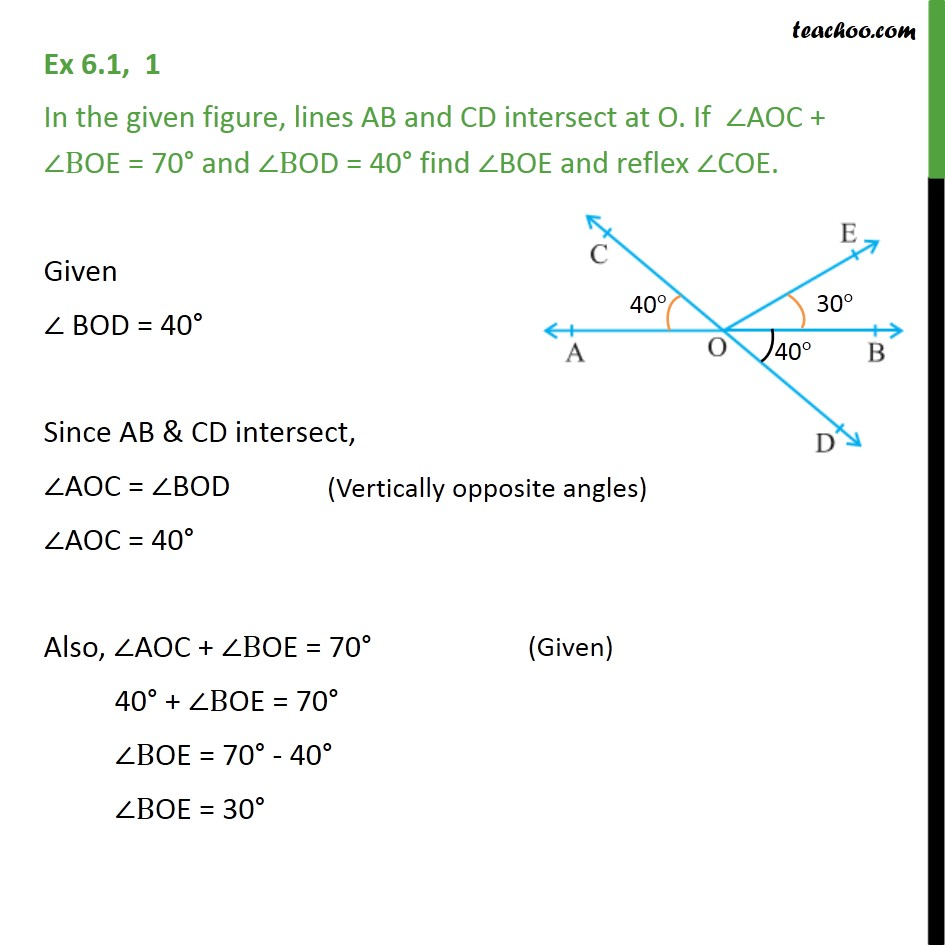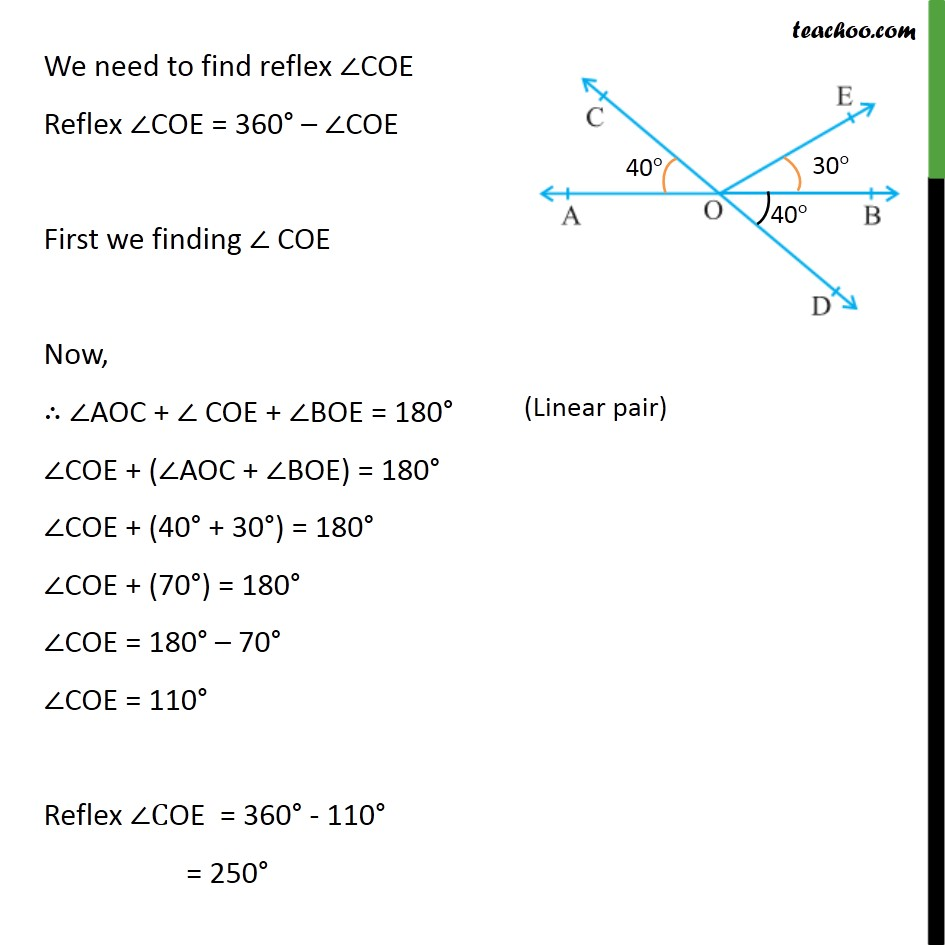1. Chapter 6 Class 9 Lines and Angles (Term 1)
2. Serial order wise
3. Ex 6.1

Transcript

Ex 6.1, 1 In the given figure, lines AB and CD intersect at O. If ∠AOC + ∠BOE = 70° and ∠BOD = 40° find ∠BOE and reflex ∠COE. Given ∠ BOD = 40° Since AB & CD intersect, ∠AOC = ∠BOD ∠AOC = 40° Also, ∠AOC + ∠BOE = 70° 40° + ∠BOE = 70° ∠BOE = 70° - 40° ∠BOE = 30° We need to find reflex ∠COE Reflex ∠COE = 360° – ∠COE First we finding ∠ COE Now, ∴ ∠AOC + ∠ COE + ∠BOE = 180° ∠COE + (∠AOC + ∠BOE) = 180° ∠COE + (40° + 30°) = 180° ∠COE + (70°) = 180° ∠COE = 180° – 70° ∠COE = 110° Reflex ∠COE = 360° - 110° = 250°